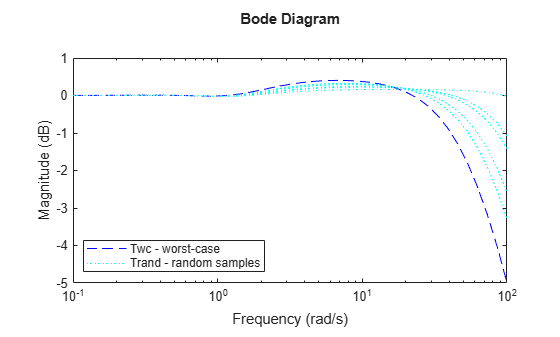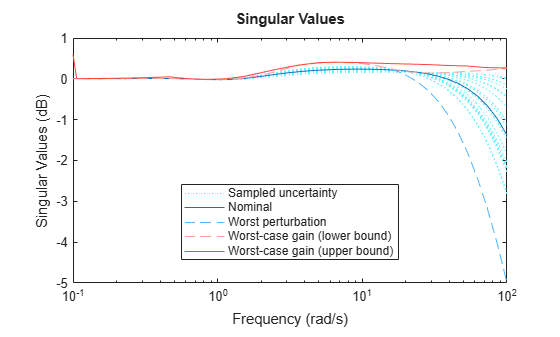Documentation

## Robust Stability and Worst-Case Gain of Uncertain System

This example shows how to calculate the robust stability and examine the worst-case gain of the closed-loop system described in System with Uncertain Parameters. The following commands construct that system.

```m1 = ureal('m1',1,'percent',20); m2 = ureal('m2',1,'percent',20); k = ureal('k',1,'percent',20); s = zpk('s'); G1 = ss(1/s^2)/m1; G2 = ss(1/s^2)/m2; F = [0;G1]*[1 -1]+[1;-1]*[0,G2]; P = lft(F,k); C = 100*ss((s+1)/(.001*s+1))^3; T = feedback(P*C,1); % Closed-loop uncertain system```

This uncertain state-space model `T` has three uncertain parameters, `k`, `m1`, and `m2`, each equal to 1±20% uncertain variation. Use `robstab` to analyze whether the closed-loop system `T` is robustly stable for all combinations of possible values of these three parameters.

```[stabmarg,wcus] = robstab(T); stabmarg```
```stabmarg = struct with fields: LowerBound: 2.8799 UpperBound: 2.8864 CriticalFrequency: 575.0339 ```

The data in the structure `stabmarg` includes bounds on the stability margin, which indicate that the control system can tolerate almost 3 times the specified uncertainty before going unstable. It is stable for all parameter variations in the specified ±20% range. The critical frequency is the frequency at which the system is closest to instability.

The structure `wcus` contains the smallest destabilization perturbation values for each uncertain element.

`wcus`
```wcus = struct with fields: k: 1.5773 m1: 0.4227 m2: 0.4227 ```

You can evaluate the uncertain model at these perturbation values using `usubs`. Examine the pole locations of that worst-case model.

```Tunst = usubs(T,wcus); damp(Tunst)```
``` Pole Damping Frequency Time Constant (rad/seconds) (seconds) -8.82e-01 + 1.55e-01i 9.85e-01 8.95e-01 1.13e+00 -8.82e-01 - 1.55e-01i 9.85e-01 8.95e-01 1.13e+00 -1.25e+00 1.00e+00 1.25e+00 7.99e-01 1.65e-06 + 5.75e+02i -2.87e-09 5.75e+02 -6.05e+05 1.65e-06 - 5.75e+02i -2.87e-09 5.75e+02 -6.05e+05 -1.50e+03 + 6.44e+02i 9.19e-01 1.63e+03 6.67e-04 -1.50e+03 - 6.44e+02i 9.19e-01 1.63e+03 6.67e-04 ```

The system contains a pair of poles very close to the imaginary axis, with a damping ratio of less than `1e-7`. This result confirms that the worst-case perturbation is just enough to destabilize the system.

Use `wcgain` to calculate the worst-case peak gain, the highest peak gain occurring within the specified uncertainty ranges.

```[wcg,wcug] = wcgain(T); wcg```
```wcg = struct with fields: LowerBound: 1.0475 UpperBound: 1.0732 CriticalFrequency: 6.9261 ```

`wcug` contains the values of the uncertain elements that cause the worst-case gain. Compute a closed-loop model with these values, and plot its frequency response along with some random samples of the uncertain system.

```Twc = usubs(T,wcug); Trand = usample(T,5); bodemag(Twc,'b--',Trand,'c:',{.1,100}); legend('Twc - worst-case','Trand - random samples','Location','SouthWest');```Alternatively use `wcsigma` to visualize the highest possible gain at each frequency, the system with the highest peak gain, and random samples of the uncertain system.

`wcsigma(T,{.1,100})`